Die and Wafer
Diodes and Rectifiers
Discrete Thyristors
IC - Power and Linear
MOSFETs
Optoelectronics
Power Modules
Capacitors
Resistors
Magnetics
Sensors
Other Components
Semiconductors
Passive Components
 My Vishay | Language

# Small Signal Diodes - Small signal switching diodes - Repetitive Peak Reverse Voltage 50 V to 100 V

Small Signal Diodes - Small signal switching diodes - Repetitive Peak Reverse Voltage 50 V to 100 V
Series
Product ImagePackage (JEDEC®) Code
VRRM
(V)
IR at VR
(nA at V)
VF at IF
(V at mA)
trr
(ns)
Circuit Configuration
AEC-Q101 QualifiedEnlarge
DO-35 (DO-204AH) 100 25 at 20 1 at 10 8 Single NoEnlarge
DO-35 (DO-204AH) 100 25 at 20 1 at 10 8 Single Yes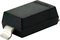Enlarge
SOD-123 100 25 at 20 1 at 10 4 Single YesEnlarge
SOD-123 100 25 at 20 1 at 10 4 Single Yes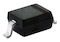Enlarge
SOD-323 100 25 at 20 1 at 10 4 Single Yes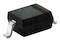Enlarge
SOD-323 100 25 at 20 1 at 10 4 Single YesEnlarge
SOD-323 100 25 at 20 1 at 10 4 Single YesEnlarge
DO-35 (DO-204AH) 50 100 at 50 0.620 at 1 4 Single Yes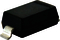Enlarge
SOD-123 50 100 at 50 0.620 at 1 4 Single Yes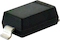Enlarge
SOD-123 50 100 at 50 0.620 at 1 4 Single YesEnlarge
DO-35 (DO-204AH) 75 50 at 50 1 at 50 4 Single NoEnlarge
SOD-123 75 50 at 50 1.0 at 50 4 Single YesEnlarge
SOD-123 75 50 at 50 1.0 at 50 4 Single Yes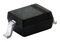Enlarge
SOD-323 75 50 at 50 1 at 50 4 Single YesEnlarge
SOD-323 75 50 at 50 1 at 50 4 Single YesEnlarge
DO-35 (DO-204AH) 100 25 at 20 0.720 at 5 8 Single NoEnlarge
SOD-123 100 25 at 20 1 at 100 4 Single YesEnlarge
SOD-123 100 25 at 20 1 at 100 4 Single Yes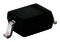Enlarge
SOD-323 100 25 at 20 1 at 100 4 Single YesEnlarge
SOD-323 100 25 at 20 1 at 100 4 Single YesEnlarge
DO-35 (DO-204AH) 100 5 at 75 1 at 10 4 Single No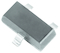Enlarge
SOT-23 70 2500 at 70 0.715 at 1 6 Single Yes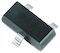Enlarge
SOT-23 70 2500 at 70 0.715 at 1 6 Single Yes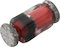Enlarge
QuadroMELF (SOD-80) 70 1 at 30 1 at 100 n/a Single No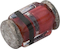Enlarge
MicroMELF 70 1 at 30 1 at 100 0 Single No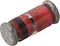Enlarge
MiniMELF (SOD-80) 70 1 at 30 1 at 100 0 Single NoEnlarge
SOT-23 75 1000 at 75 0.715 at 1 6 Single YesEnlarge
SOT-23 75 1000 at 75 0.715 at 1 6 Single YesEnlarge
SOD-123 100 1000 at 75 1.25 at 150 6 Single YesEnlarge
SOD-123 100 1000 at 75 1.25 at 150 6 Single YesEnlarge
SOD-323 100 1000 at 75 1.250 at 150 6 Single YesEnlarge
SOD-323 100 1000 at 75 1.250 at 150 6 Single YesEnlarge
DO-35 (DO-204AH) 70 1 at 30 1 at 100 0 Single Yes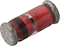Enlarge
MiniMELF (SOD-80) 60 100 at 50 1 at 100 50 Single YesEnlarge
DO-35 (DO-204AH) 60 100 at 50 1 at 100 50 Single Yes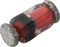Enlarge
QuadroMELF (SOD-80) 60 100 at 50 1 at 100 50 Single No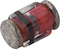Enlarge
MicroMELF 60 100 at 50 1 at 100 50 Single YesEnlarge
SOT-23 70 2500 at 70 0.715 at 1 6 Common cathode YesEnlarge
SOT-23 70 2500 at 70 0.715 at 1 6 Common cathode YesEnlarge
SOT-23 70 2500 at 70 0.715 at 1 6 Dual serial YesEnlarge
DO-35 (DO-204AH) 75 100 at 60 0.750 at 10 6 Single YesEnlarge
SOT-23 70 2500 at 70 0.715 at 1 6 Common anode YesEnlarge
SOT-23 70 2500 at 70 0.715 at 1 6 Common anode YesEnlarge
DO-35 (DO-204AH) 75 100 at 50 1 at 100 4 Single NoEnlarge
SOT-23 75 2500 at 70 1.0 at 10 4 Single Yes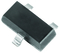Enlarge
SOT-23 75 2500 at 70 1.0 at 10 4 Single YesEnlarge
SOT-23 75 2500 at 70 0.72 at 5 4 Single YesEnlarge
SOT-23 75 2500 at 70 0.72 at 5 4 Single YesEnlarge
MiniMELF (SOD-80) 100 25 at 20 1 at 50 8 Single NoEnlarge
MiniMELF (SOD-80) 100 25 at 20 1 at 50 8 Single NoEnlarge
MiniMELF (SOD-80) 50 100 at 50 0.620 at 1 4 Single YesEnlarge
MiniMELF (SOD-80) 50 100 at 50 0.620 at 1 4 Single YesEnlarge
MiniMELF (SOD-80) 75 50 at 50 1 at 50 4 Single NoEnlarge
MiniMELF (SOD-80) 75 50 at 50 1 at 50 4 Single NoEnlarge
MiniMELF (SOD-80) 100 25 at 20 0.720 at 5 8 Single No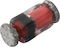Enlarge
QuadroMELF (SOD-80) 100 25 at 20 1 at 50 8 Single NoEnlarge
QuadroMELF (SOD-80) 50 100 at 50 0.620 at 1 4 Single YesEnlarge
QuadroMELF (SOD-80) 75 50 at 50 1 at 50 4 Single NoEnlarge
QuadroMELF (SOD-80) 100 25 at 20 0.720 at 5 8 Single No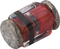Enlarge
MicroMELF 100 25 at 20 1 at 50 8 Single NoEnlarge
MicroMELF 75 50 at 50 1 at 50 4 Single NoEnlarge
MicroMELF 100 25 at 20 0.720 at 5 8 Single NoEnlarge
SOT-23 70 100 at 50 0.7 at 1 4 Single YesEnlarge
SOT-23 100 1000 at 50 0.70 at 1 4 Dual serial YesEnlarge
SOT-23 100 1000 at 50 0.70 at 1 4 Dual serial YesEnlarge
SOT-23 100 25 at 20 1 at 10 4 Single YesEnlarge
SOT-23 100 25 at 20 1 at 10 4 Single Yes